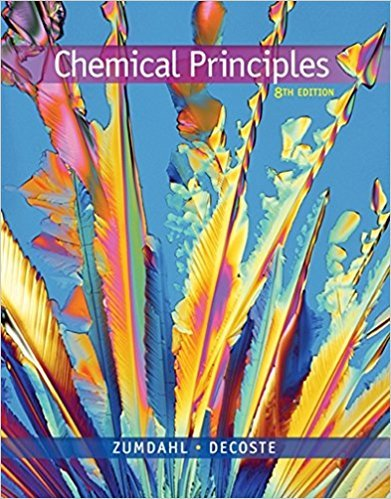×
Get Full Access to Chemical Principles - 8 Edition - Chapter 9 - Problem 9.126
Get Full Access to Chemical Principles - 8 Edition - Chapter 9 - Problem 9.126

×

# Consider a sample containing 5.00 moles of a monatomicISBN: 9781305581982 176

## Solution for problem 9.126 Chapter 9

Chemical Principles | 8th Edition

• Textbook Solutions
• 2901 Step-by-step solutions solved by professors and subject experts
• Get 24/7 help from StudySoup virtual teaching assistantsChemical Principles | 8th Edition

4 5 1 332 Reviews
13
2
Problem 9.126

Consider a sample containing 5.00 moles of a monatomic ideal gas that is taken from state A to state B by the following two pathways: Pathway one: PA 5 3.00 atm 1 PC 5 3.00 atm VA 5 15.0 L 88n VC 5 55.0 L 2 PB 5 6.00 atm 88n VB 5 20.0 L Pathway two: PA 5 3.00 atm 3 PD 5 6.00 atm VA 5 15.0 L 88n VD 5 15.0 L 4 PB 5 6.00 atm 88n VB 5 20.0 L For each step, assume that the external pressure is constant and equals the final pressure of the gas for that step. Calculate q, w, DE, and DH for each step, and calculate overall values for each pathway. Explain how the overall values for the two pathways illustrate that DE and DH are state functions, whereas q and w are path functions.

Step-by-Step Solution:
Step 1 of 3

Week of 2/29- 3/4 ENERGY IN CHEMISTRY Energy is the ability to do work. It is neither created nor destroyed, simply transferred. Kinetic energy and potential energy are present in chemical bonds SOURCES OF KINETIC ENERGY IN CHEMICAL SYSTEM -particles move and rotate through space -bonds vibrate -electrons move around nucleus SOURCES OF POTENTIAL ENERGY IN CHEMICAL SYSTEM -bonded atoms attract and repel -the attraction between nucleus and electrons, also the repulsion between electrons and electrons - Attraction b/w nucleus and shared electrons During chemical reactions, change occurs in BONDING - Changes in the way atoms are bonded involve changes in chemical potential energy - System- the reaction itself - Surroundings- imme

Step 2 of 3

Step 3 of 3

##### ISBN: 9781305581982

The answer to “Consider a sample containing 5.00 moles of a monatomic ideal gas that is taken from state A to state B by the following two pathways: Pathway one: PA 5 3.00 atm 1 PC 5 3.00 atm VA 5 15.0 L 88n VC 5 55.0 L 2 PB 5 6.00 atm 88n VB 5 20.0 L Pathway two: PA 5 3.00 atm 3 PD 5 6.00 atm VA 5 15.0 L 88n VD 5 15.0 L 4 PB 5 6.00 atm 88n VB 5 20.0 L For each step, assume that the external pressure is constant and equals the final pressure of the gas for that step. Calculate q, w, DE, and DH for each step, and calculate overall values for each pathway. Explain how the overall values for the two pathways illustrate that DE and DH are state functions, whereas q and w are path functions.” is broken down into a number of easy to follow steps, and 146 words. This full solution covers the following key subjects: . This expansive textbook survival guide covers 20 chapters, and 2754 solutions. The full step-by-step solution to problem: 9.126 from chapter: 9 was answered by , our top Chemistry solution expert on 12/23/17, 04:53PM. This textbook survival guide was created for the textbook: Chemical Principles, edition: 8. Chemical Principles was written by and is associated to the ISBN: 9781305581982. Since the solution to 9.126 from 9 chapter was answered, more than 289 students have viewed the full step-by-step answer.

Unlock Textbook Solution